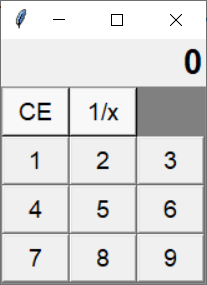TORONTO KIDS COMPUTER CLUB | Markham Saturday 9:30 Python Homework 20.06.13.
17696

# Markham Saturday 9:30 Python Homework 20.06.13.

## 17 Jun Markham Saturday 9:30 Python Homework 20.06.13.

Question：

Continue to program the code we made in the class at the bottom of this page. Try to use for loop to create buttons from 1-9, so the tkinter window would display as below:Hint:
You can use “for i in range(1, 10):” or “for i in ‘123456789’:”  to build these 9 buttons, however, you should thinking of how to use math techniques to solve the row and column.

for example, buttons 1,2,3 are in row 1 and buttons 4,5,6 are in row 2. You should think that int(1/3) is 0 or 1//3 is 0,  2//3 is still 0, 3//3 is 1, 4//3 is 1, 5//3 is 1, 6//3 is 2. Then how can you make 1,2,3 all become 1, while 4,5,6 all become 2?

You should use %(finding division remainder) to solve columns, but how?

Code:

`from tkinter import *class Calculator:    def __init__(self, master):        self.master = master        master.title('Calculator')        self.f1 = Frame(master)        self.f1.option_add('*Font', 'arial 20 bold')        self.f1.pack(expand=YES, fill=BOTH)        self.strResult = StringVar()        self.strResult.set('0')        self.lblResult = Label(self.f1, textvariable=self.strResult, justify='right')        self.lblResult.pack(side=RIGHT)        self.f2 = Frame(master, borderwidth=5, bd=1, bg='grey')        self.f2.option_add('*Font', 'arial 14')        self.f2.pack(side=TOP, expand=YES, fill=BOTH)        self.btnCE = Button(self.f2, text='CE', bg='#f8f8f8', width=4, command=self.reset)        self.btnCE.grid(row=0, column=0)        # reciprocal 3/7 -> 7/3         self.btnRecip = Button(self.f2, text='1/x', bg='#f8f8f8', width=4, command=self.recip)        self.btnRecip.grid(row=0, column=1)    def reset(self):        pass    def recip(self):        passtk = Tk()myCal = Calculator(tk)tk.mainloop()`